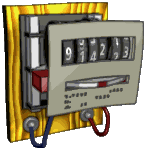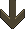# Electricity cost calculator kW/h

 This calculator enables you to find out the cost of running an electric appliance for a specific amount of time. All you need to know is the power the device consumes in watts and the rate you pay for your electricity. The rate is usually found on your electricity bill and will be stated in pence per kWh. Eg: My rate is currently 22.19 pence per kWh. Input three values of your own and click the ‘Calculate cost’ button. This will work for any currency. The rate currency drop down box only changes the symbol before the calculated cost.Enter the appliance power: WattskW Enter the time the appliance is on: MinutesHoursDaysWeeksMonthsQuartersYearsDecadesCenturies Enter your electricity rate: GBP – pence/kWhUSD – cents/kWhEUR – cents/kWhAUD – cents/kWhOtherAppliance running cost: £ Appliance running cost (Rounded, ideal for small values): £ Power in kWh: kWh

 The maths behind the calculator A mixture of the formulas below and more are used depending on what units you select above. The simple calculation is the rate multiplied by the watts (in kW) gives us the cost for one hour. cost in pence per hour = Rate x kW To use watts instead of kW, we divide the watts by 1000. cost in pence per hour = (Watts ÷ 1000) x Rate To get the cost for 1 minute, we divide the cost per hour by 60. cost in pence per minute = (Watts ÷ 1000) x Rate ÷ 60 To get the cost for 1 day, we multiply the cost per hour by 24. cost in pence per day = (Watts ÷ 1000) x Rate x 24 To get the cost for 1 week, we multiply the cost per hour by 168. cost in pence per week = (Watts ÷ 1000) x Rate x 168 To make the answer into pounds, we divide the pence by 100 and round it up to two decimal places. cost in pounds per hour = (Watts ÷ 1000) x Rate ÷ 100 To get the cost in pounds for 1 week, we multiply the cost per hour in pounds by 168. cost in pounds per week = (Watts ÷ 1000) x Rate x 168 ÷ 100 To get the power in kWh, we take the watts in kW and multiply it by the time in hours. power in kWh = kW x time in hours